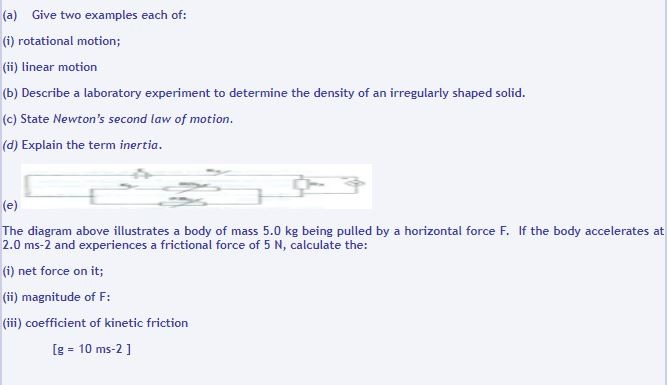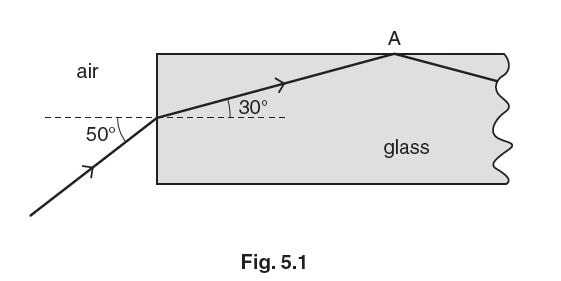# WAEC Physics Questions For Essay And Objective

WAEC Physics Questions For Essay And Objective

PAPER 2 [Essay]

1. A particle is projected horizontally at 15ms-1 from a height of 20m.
Calculate the horizontal distance covered by the particle just before hitting the ground.
[g = 10 ms-2]

ANS: Let R represent the horizontal distance covered at time, t.
h = ½ gt2
20 = ½ x 10 x t2
t = 2s

R  = ut

=  15 x 2

= 30m

2. (a) tate Faraday’s second law of electrolysis.

(b) An electric charge of 9.6 x 104 C liberates 1 mole of substance containing 6.0 x 1023 atoms.  Determine the value of the electronic charge.

ANS: (a) If the same quantity of electricity is passed through different  voltameters/electrolytes connected in series the masses of the substances liberated/deposited during electrolysis is (directly) proportional to their chemical equivalents.

(b) Let e represent the electronic charge.

=          9.6 x 104
6. 0. x 1023

=  1.60 x 10-19C

3. State three methods of polarizing an unpolarized light.

4. (a) State Faraday’s second law of electrolysis.

(b) An electric charge of 9.6 x 104 C liberates 1 mole of substance containing 6.0 x 1023 atoms. Determine the value of the electronic charge.

ANS: (a) The mass of an element deposited /liberated during electrolysis is (directly) proportional to the chemical equivalent of the element.

(b) Let e represent the electronic charge.

=  9.6 x104
6. 0. x 1023

=  1.60 x 10-19C

5. Explain the following terms:
(a) tensile stress;

(b) Young’s modulus.

6. (a) Define diffusion.
(b) State two applications of electrical conduction through gases.

ANS: (a) Diffusion is the process by which substances mix with one another due to the random motion of their molecules.

(b) Applications of electrical conduction through gases include:

– In advertising industry/ Neon signs
– In lighting/ fluorescent tubes

– Identification of gases
– Cathode ray oscilloscope/ T.V. tubes.

7. (a) List two properties of cathode rays.

(b) Explain how the intensity and energy of cathode rays may be increased.
ANS: (a) Properties of cathode rays:

– They are negatively charged.
– travel in straight line in field free space.

– are deflected by electric/magnetic field.
– possess (kinetic) energy.

– possess momentum.
(b) The intensity of cathode rays may be increased by raising the  temperature of the cathode /increasing the current through the heater.

They may be increased by raising the potential difference between the anode and the cathode/ the anode potential.

8. Give three observations in support of de Broglie’s assumption that moving particles behave like waves.

9. (a) Differentiate between plane polarization and interference as applied to waves.
(b) List two uses of polaroids.

ANS: (b) They are used in:
– production of 3-dimentional films

– determination of concentration of sugar solution
– polaroid cameras

– sun glasses.

10. (a) State two factors which affect the mass of elements deposited during electrolysis.
(b) List two non-electrolytes.

ANS: (a) time, current /quantity of charge, nature/ e.c.e of element.
(b) water, kerosene, petrol, benzene, ethanol.

11.12. (a) i. State two advantages of alcohol over mercury as a thermometric liquid.

ii. When the bulb of a thermometer is placed in a beaker of hot water, the level of mercury first falls and then rises gradually. Explain this observation.

(b) List two factors, other than temperature, that affect the rate of evaporation of a liquid.

(c) A block of lead of mass 100kg in a crucible and at a temperature of 40oC was placed in an electric furnace rated 10kW. If the melting point of lead is 320oC, calculate the:

(i) quantity of heat required to heat the lead to its melting point;

(iii) time taken to supply this additional energy.
(Specific heat capacity of lead = 120JKg-1 K-1

(Specific latent heat of fusion of lead = 2.5 x 104 JK-1)

(d) State two precautions necessary in an experiment to determine the specific latent heat of vaporization of water.

ANS: (a) i. Advantages of alcohol over mercury as a thermometric liquid
–  lower freezing point

–  cheaper
– easily expansively.

ii. the glass of the bulb of the thermometer first expands; hence the mercury level fails. The expansion of the mercury increases more than that of the glass and the liquid rises.

(b) Factors other than temperature affecting the rate of evaporation of a liquid
– wind
– humidity/ dryness of air

–  nature of liquid/ viscosity /density
– area of liquid exposed

–  pressure.

13. (a) State two factors which affect the angle of deviation of a ray of light through a triangular glass prism.

(b) Seven virtual images of an object are formed when two plane mirrors are inclined at an angle 0 to each other. Calculate the value of 0.

(c) By means of a ripple tank, a student was able to generate series of transverse waves by varying the frequency ofthe dipper and all the waves so generated covered a distance of 0.80 m in 0.2 s.

(i) Determine the speed, v, of the waves
(ii) Copy and complete the table given above in your answer booklet.

(iii) Plot a graph with f on the vertical axis and h -1 on the horizontal axis.
(iv) What does the slope of the graph represent?

14. The Fig. 5.1 below shows a ray of light entering and passing along an optical fibre.(a) Calculate the refractive index of the glass in the optical fibre.

(b) Explain why the ray of light is totally internally reflected at A.

(c) Both optical fibre and copper wire are used to transmit data. Optical fibre is cheaper and can carry more data per second than copper wire.

State one other advantage of using optical fibre rather than copper wire to transmit data.

15. (a) State two differences between a sound wave and a radio wave

(b) Explain why a vibrating tuning fork sounds louder when its stem is pressed against a table top than when held in air.

(c) State two conditions necessary for the:
i. production of stationary wave in a medium;

ii. formation of interference wave patterns;

iii. occurrence of total internal reflection of a wave.

(d) i. A ray of light is incident on one face of an equilateral glass prism.Draw a ray diagram to show the path of the ray through the prism.

ii. Calculate the refractive index of the glass if the angle of minimum deviation is 41o.

Paper 1 [Objective]

Answer ALL questions in this section.

1. An electron moves with a speed of 2.00 x 107 ms-1 in an orbit in a uniform magnetic field of 1.20 x 10-3T. Calculate the radius of the orbit.

[Mass of an electron = 9.11 x 10-31kg; charge on an electron = 1.61 x 10-19C]

2. A tennis ball projected at an angle attains a range R = 78 m. If the velocity imparted to the ball by the racket is 30ms-1, calculate.

[g=10ms-2]

3. A metallic bar 50 cm long has a uniform cross-sectional area of 4.0 cm2.  If a tensile force of 35kN produces an extension of 0.25 mm, calculate the value of Young’s modulus.

4. A stone projected horizontally from the top of a tower with a speed of 4 ms-1 lands on the level ground at a horizontal distance of 25 m from the foot of the tower. Calculate the height of the tower.
[g = 10 ms-2]

5. A spiral spring with a metal extends by 10.5cm in air. When the metal is fully in water, the spring extends by 6.8 cm. Calculate the relative density of the metal (Assume Hooke s law Is obeyed)

6. A ray of light is incident on an air-glass boundary at an angle t? If the angle between the partial reflected ray and the refracted ray is 90°, calculate θ given that the refractive index of glass is 1.50.

7. A ball is projected horizontally from a height with a velocity of 40 ms-1. Calculate the drop in height after travelling a horizontal distance of 30m.

[Neglect air resistance; g = 10 ms-1].